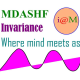# Relativistic Doppler effect

This lecture was delivered to the final year honors class of 3 year science degree students on 15 November 2017 as part of the Classical Dynamics paper.

### Relativistic Doppler effect

There is an apparent shift in the observed frequency of any electromagnetic wave ( light ) when there is any relative motion between the source of light and the observer. This can be easily determined by using the 4-vector formulation of theory of relativity.

Lets discuss the details of this phenomena under two situations.

### A. Source is at rest and observer is in motion

Lets us consider two inertial frames S and S’. S’ is moving wrt S, along the x-axis with speed$v = \beta c$ where the observer is at rest in S’ frame but the source is at rest in the  S frame.Relativistic Doppler effect: the source is at rest in unprimed frame S and the observer is at rest in the primed frame, that is, S’ frame. Photo Credit: mdashf.org

Let the source emit a radiation of energy$E = h {\nu}_0$, which makes an angle$\theta$ wrt x-axis. So the momentum is given by$p = \frac{E}{c}=\frac{h\nu_0}{c}$. The$p_x$ component of the momentum is therefore$p_x=p \cos \theta = \frac{h\nu_0}{c} \cos\theta$. Lets consider the x, y, z and t components through the indices 1, 2, 3 and 0. i.e. x ≡ 1, y ≡ 2 ,z ≡ 3 and t ≡ 0.

Then the Lorentz transformation for the 4-momentum$p^{\mu}$ is given by:$\boxed{p^{\mu}{'}=\Lambda^{\mu}_{\nu}\, p^{\nu}}$ where$p^{\mu}{'}$ is the contravariant 4-vector in S’ frame and$p^{\nu}$ is the same in the S frame.$\Lambda^{\mu}_{\nu}$ is the Lorentz transformation matrix element$\mu$ for row and$\nu$ for column.$p^{\mu}{'} \equiv ({p^0}', \,{p^1}',\, {p^2}',\, {p^3}')=(\frac{h\nu_0}{c}, \,p_x, \,p_y, \, p_z )$.$\vec{p}=(p_x,\,p_y,\,p_z)$ is the 3-momentum.$\Lambda^{\mu}_{\nu} = \begin{pmatrix}\gamma & - \gamma \beta & 0 & 0\\ - \gamma \beta & \gamma & 0 &0 \\0 & 0 & 1 &0 \\0 & 0 & 0 & 1\end{pmatrix}$ so that$\begin{pmatrix} {p^0}' \\ {p^1}' \\ {p^2}' \\ {p^3}'\end{pmatrix} = \begin{pmatrix}\gamma & - \gamma \beta & 0 & 0\\ - \gamma \beta & \gamma & 0 &0 \\0 & 0 & 1 &0 \\0 & 0 & 0 & 1\end{pmatrix}=\begin{pmatrix} p^0 \\ p^1 \\ p^2 \\ p^3 \end{pmatrix}$.

The “time” or zeroth component is then${p^0}'=\gamma(p^0 - \beta p^1)$, where$p^1=p_x =p \cos \theta=\frac{h \nu_0}{c} \cos \theta$.

So that;$\frac{h {\nu}\,'}{c}= \gamma (\frac{h \nu_0 }{c}- \beta \frac{h \nu_0 }{c} \cos \theta) = \gamma \frac{h \nu_0 }{c}(1- \beta \cos \theta)$.

So,$\boxed{\nu\,' = \frac{\nu_0 1- \beta \cos \theta)}{\sqrt{1-\beta^2}}}$.

Thus observed frequency depends on angle$\theta$ and motion of observer.

There are 3 situations to discuss.

#### Case i

Light is emitted at$\theta =0^0$.

Motion of observer is to the right — i.e. receding observer. So$\cos \theta =1$.$\nu \,' = \frac{\nu_0 (1-\beta)}{\sqrt{1-\beta^2}} =\nu_0 \sqrt{\frac{1-\beta}{1+\beta}}$.

So$\boxed{\nu \,' = \nu_0 \sqrt{\frac{1-\beta}{1+\beta}}}$$\nu \,' < \nu_0$.

#### Case ii

Light emitted at an angle$\theta = \pi$ as S’ moves towards source.$\cos \theta =-1$.$\nu \,' = \frac{\nu_0 (1+\beta)}{\sqrt{1-\beta^2}} =\nu_0 \sqrt{\frac{1+\beta}{1-\beta}}$.

So$\boxed{\nu \,' =\nu_0 \sqrt{\frac{1+\beta}{1-\beta}}}$$\nu \,' > \nu_0$.

Case i and ii are known as longitudinal Doppler effect.

#### Case iii

Light is emitted at an angle$\theta = 90^0$, observer is moving towards source.

So,$\boxed{\nu \,' = \frac{\nu_0}{\sqrt{1-\beta^2}}}$.

Case iii is known as transverse Doppler effect.

### B. Observer is at rest and source is moving

Lets consider an observer to be at rest in the S frame while the source is at rest in the S’ frame and S’ frame is moving wrt S frame with speed given by$\beta = \frac{v}{c}$, as before.

We need to find the inverse transformation:$p = \Lambda ^{-1} p\,'$ where$\Lambda^{-1} = \begin{pmatrix}\gamma & \gamma \beta & 0 & 0\\ \gamma \beta & \gamma & 0 &0 \\0 & 0 & 1 &0 \\0 & 0 & 0 & 1\end{pmatrix}$ and$\begin{pmatrix} p^0 \\ p^1 \\ p^2 \\ p^3 \end{pmatrix} = \begin{pmatrix} \gamma & \gamma \beta & 0 & 0\\ \gamma \beta & \gamma & 0 &0 \\0 & 0 & 1 &0 \\0 & 0 & 0 & 1\end{pmatrix} = \begin{pmatrix} {p^0}' \\ {p^1}' \\ {p^2}' \\ {p^3}'\end{pmatrix}$.

Now time component is:$p^0 = \gamma (p^0\hspace{1pt}'+\beta p^1 \,')$$\frac{h\nu}{c} = \gamma (\frac{h\nu_0}{c} + \beta \frac{h\nu_0}{c} \cos \theta \,')= \gamma \frac{h\nu_0}{c}(1+\beta \cos \theta \,')$.

So,$\boxed{\nu = \frac{\nu_0 (1+\beta \cos \theta \,')}{\sqrt{1-\beta^2}}}$ where

•$\nu$: observed frequency — in unprimed, i.e. S-frame.
•$\nu_0$: emitted frequency — in primed, i.e. S’-frame.
•$\theta \,'$: angle made by emitted light with x’ axis, which is parallel to x-axis.

As before there are 3 situations to discuss specially.

#### Case i. source is approaching observer,$\theta \,' = 0$$\nu = \frac{\nu_0(1+\beta)}{\sqrt{1-\beta^2}} \,'$.$\boxed{\nu = \nu_0 \sqrt{\frac{1+\beta}{1-\beta}}}$.$\nu \,> \nu_0$.

#### Case ii. source is receding away from observer,$\theta \, ' = \pi$.$\nu = \frac{\nu_0(1-\beta)}{\sqrt{1-\beta^2}} \,'$.$\boxed{\nu = \nu_0\sqrt{\frac{1-\beta}{1+\beta}}}$.$\nu \, < \nu_0$.

#### Case iii. source is in transverse motion wrt observer,$\theta \,' = 0$.$\boxed{\nu= \frac{\nu_0}{\sqrt{1-\beta^2}}}$.

i and ii are known as longitudinal Doppler effect and iii is known as transverse Doppler effect as before.

We see that for relative approach of source and observer apparent or observed frequency increases. Its known as BLUE SHIFT.

Similarly for recession between source and observer there is apparent decrease in source frequency known as RED SHIFT.

#### TagsI am an experimental particle physicist, traveler, teacher, researcher, scientist and communicator of ideas. I am a quarkist and a bit quirky ! Hypothesis non fingo, eppur si muove, dubito cogito ergo sum are things that turn me on ! Researcher in experimental high energy physics (aka elementary particle physics; like “quarks, leptons & mesons and baryons”) … Teacher of Physics (and occasionally chemistry and maths) Blogger (check my website; mdashf.org) ! Love to read read and read but only stuff that interest me. Love to puff away my time in frivolities, just dreaming and may be thinking. Right now desperately trying to streamline myself.